﻿ The Effect of Load Modeling on Load Flow Results in Distribution SystemsPublications are Open
Access in this journal
Article Versions
Export Article
• Normal Style
• MLA Style
• APA Style
• Chicago Style
Original Article
Open Access Peer-reviewed

### The Effect of Load Modeling on Load Flow Results in Distribution Systems

Nour ali Rostami, Mahmoud Oukati Sadegh
American Journal of Electrical and Electronic Engineering. 2018, 6(1), 16-27. DOI: 10.12691/ajeee-6-1-3
Published online: February 03, 2018

### Abstract

Effective utilization of power distribution networks requires extensive studies in such areas as using of capacitors, voltage regulators, network reconfiguration, and so on. Indeed, achieving to accurate answers, and managing appropriate solutions for network problems requires a detailed modeling of the network in the process of the above studies. Among the elements that are important for modeling in network research is network loads. Loads are generally being modeled such as constant power. While load nature is often widespread and different. Failure to have a detailed modeling can lead to non-optimal and even wrong answers, and will result in waste of costs and investments. Since the load flow is the basis of any research in distribution networks, in this paper the effect of load modeling on load flow results which can clarify the importance of the load modeling for other network studies are investigated. What can be inferred from this study is that any study in distribution networks can only lead to optimal results if the load model is accurate. Therefore, attempting to measure necessary load parameters and using their exact modeling methods in distribution networks should be done in order to obtain more accurate results and more savings.

### 1. Introduction

Load flow is a basis of many studies in power systems. In distribution network studies such as design, development, optimization issues, etc., load flow is one of the basic tools, and therefore requires a simple and reliable way to carry out the load flow. Over the past several decades, effective load flow methods such as the Gauss-Seidel, Newton-Raphson, and Fast Decoupled 1, 2, 3 have been developed and widely used for power control, and design of power systems. Although these load flow methods are simple, they cannot be used effectively to analyze radial distribution systems. Gauss-Seidel, Newton-Raphson, and Fast Decoupled methods have been developed for high-voltage networks. In these methods, by increasing the R/X ratio of lines, the convergence rate is greatly reduced, and it is even possible not to find the answer in networks with R and X lines close together. The Newton Raphson and Fast Decoupled methods can solve most of the normal networks with high efficiency, but in radial distribution networks due to the wide range of resistance and reactance, as well as radial structure, do not work well.

Researchers have modified the Newton-Raphson and Fast Decoupled Load flow methods several times 4, 5. It has been shown repeatedly that these methods may be inefficient in analyzing power systems with high R/X ratio or specific network structures, and for weak power systems there is no convergence 4, 6.

In 7, attempts have been made using a ladder network theory to analyze the load flow of a radial distribution network. Stevens et al. 8 have shown that The ladder-based method is fast, but in some cases, not convergent.

In 9, a different method for solving the load flow problem involving two steps is suggested. In the first step, all buses located outside of each branch are identified, and in the next step, the current of branches and bus voltages are calculated. But in a large power system, a long time is required to identify the buses, and therefore algorithm does not have a high speed. Shirmohammadi 10 has presented a compensation-based load flow method for radial distribution networks or weak meshed structures. The radial section has been solved using a two-stage straightforward process in which the current of branches has been calculated at first (backward sweep), then the bus voltage is updated (forward sweep).

11, 12 have proposed a load flow system that uses the Bus Injection-to-Branch Current (BIBC) and Branch Current-to-Bus Voltage (BCBV) matrices. But this does not work for large networks. 13 has been investigated the effect of the voltage dependence load on the results of the load flow. In 14, a load flow method has been proposed for voltage-dependent load models. In the proposed method, the parallel capacity of the line and static load combinations are considered in the solution of the load flow. This method is based on updating the backward and forward voltage using the polynomial voltage equation for each branch and the backward ladder equation (Kirchhoff law). This method has been investigated for different loading conditions, different R/X ratios and different voltage levels under a wide range of load combinations. This method has a fast and acceptable convergence.

One of the easiest and most efficient ways of the load flow, which is widely used in radial distribution networks, is the backward-forward sweep technique that its convergence speed is very high. The proper operation of the power network in a steady state and the equalization of the bus voltage values with their nominal values depend on the analysis of the changes in consumption. Therefore, the precise description of the load is very important. To carry out load flow correctly and loss studies in the distribution network, the load model and line model play a valuable role. In load flow and other distribution network studies, the constant power model is considered as load model, Since the distribution network has loads depending on the power, current and / or impedance, or a mixture of them, the constant power model cannot be a suitable, effective and accurate model for the load and it may even lead to inaccurate results. In this paper, the effect of load models on the results of load flow is investigated. The purpose of the study is to investigate the effect of load modeling on distribution network studies. In the following, load models are introduced and then the method of solving backward-forward sweep load flow is presented, after that, the effect of the load models on losses and voltage profile in distribution networks will be reviewed. Two networks are selected as prototypes, the load flow of two networks and the results of active power loss, reactive power consumption and voltage profile for different load models have been presented.

### 2. Load Model

The load model is a set of algebraic equations that describes the relationship between voltage bus (amplitude and frequency) and load (active and reactive power). There are two general models for estimating load parameters, the first model is the static load model, which is most commonly used for load flow problems and calculating line power loss and other network operations in steady state. The second model is the dynamic load model, which is usually used to study the dynamics and stability of the network, regulating the relays, and all those that depend on the dynamic of network and load in the transient state. This paper focuses on static load model that is suitable for load flow problems.

2.1. Static Load Model

The static model of the load expresses the voltage and frequency relationships with active and reactive power in a momentary flow vs. time. Static load models are used to display static load combinations, such as resistance and light loads, and as an approximation for dynamic loads such as induction motors 15. In general, there are two static load models: the exponential model and the polynomial load model.

2.1.1. Exponential Load Model

The static load model expresses the load characteristics at any moment in time as algebraic functions in terms of the bus voltage magnitude and frequency at the moment. The active power component (P) and the reactive power component (Q) are considered separately.

The dependence of load characteristics on the voltage is usually shown as follows: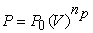(1)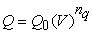(2)

P and Q are active and reactive powers in the voltage range V, and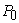and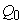are power values in the initial working conditions. The parameters of this model are np and nq. The usual values for the np and nq are given in Table 1 14. The evaluation of the np and nq requires field measurements and the parameter estimation method 16. If these indexes are zero, the model represents a constant power load model, and if the indexes are equal to one, the model represents a constant current load model that power varies directly with the voltage magnitude. If these load indexes are equal to two, the model represents a constant impedance model that can be directly varied by the square voltage magnitude.

In combination loads that combine dynamic and static load models, the index values depend on the characteristics of the combined load components. The np and nq indexes are equal to the slope of dP/dV (or dQ/dV) at V = V0. In combined loads, np is usually between 0.5 to 1.8 and nq is between 1.5 to 6. An important characteristic of nq is that it changes nonlinearly with voltage, due to magnetic saturation in distribution transformers and motors. At higher voltages, Q is greatly increased. If there is no data, the most common method is to simulate the active power load as a constant current (np = 1) and reactive power load as a constant impedance (nq = 2) 17.

2.1.2. Polynomial Load Model

In power systems, there are different loads such as residential, commercial and industrial loads. Generally, there are three types of industrial, residential and commercial load per node. The share of each load in total load varies over time, as shown in Table 2. α, β and γ are the percentages of residential, commercial and industrial load per node, respectively. Therefore, the voltage-dependent load model can be expressed as follows 16.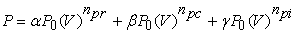(3)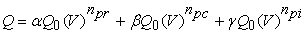(4)(5)

npr and nqr: Active and reactive load power indexes in residential loads,

npc and nqc: Active and reactive load power indexes in commercial loads,

npi and nqi: Active and reactive power load in industrial loads.

One of the polynomial models widely used in equations and networks is the ZIP model. This model is called ZIP because it is a combination of constant power, constant current and constant impedance models.

This model is displayed as 13.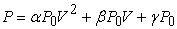(6)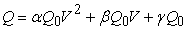(7)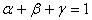(8)

α, β and γ are the percentage of the contribution of constant impedance, constant current and constant power load in each node, respectively. In the equations, active and reactive power is only investigated due to voltage variations, and the variation of power due to the frequency is not considered.andare the active and reactive power of the primary work points. If the frequency dependent parameter is also considered, power equations are written as 16.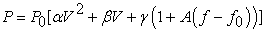(9)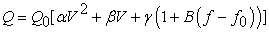(10)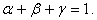(11)

In these equations A and B determine the load sensitivity to the frequency variations of the nominal value, f is the frequency at the voltage V and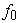is the nominal frequency. If frequency dependence is not considered (A = B = 0), the same polynomial model of ZIP is obtained. Due to the low frequency range variations in the distribution network, frequency dependencies of loads are often ignored 16.

### 3. Load Flow of Backward-forward Sweep

This load flow method consists of two stages of backward-forward sweep. The backward sweep is used to calculate the current of lines and the forward sweep is used to calculate the bus voltage. In conventional load flow studies, it is assumed that active and reactive powers is constant regardless of the voltage range in their corresponding bus. In fact, there are different types of loads, such as residential, commercial and industrial, in power system. The nature of these loads is such that their active and reactive power is dependent on voltage and frequency of the system. In addition, load characteristics have important effects on the solution of load flow and convergence capability 9. The structure of distribution systems is more like a tree with several lateral and lateral branches. It is assumed that the radial distribution network is symmetric. The single-line graph of a radial distribution feeder is shown in Figure 1. The number of branches between the sending buses and receiving buses of this feeder is shown in Table 3.

• Figure 1. Single line diagram of radial distribution feeder

By considering branch 1, the bus voltage of the receiver side is calculated as follows: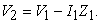(12)

Similarly, for branch 2 we will have: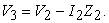(13)

In this feeder, since the bus 1 is the source, the voltage V1 is known. Therefore, having I1 and the Z1, the voltage V2 can be calculated. Voltage V3 can also be calculated with I2, Z2 and V2. Similarly, the voltage of the remaining buses is also calculated by the current of the branches.

The flow of the branch 1 is equal to the total load currents of all buses and the total capacitive currents of all buses after branch 1.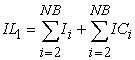(14)

NB is the number of buses. The total number of branches is equal to the number of buses minus one. So the number of branches will be equal to LN = NB-1. Similarly, the flow of the branch 2 is equal to the total load of all buses after branch 2 and with the total capacitive currents of all buses after branch 2.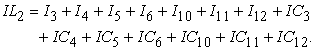(15)

The load current in the bus i is calculated as follows.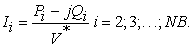(16)

The capacitive current at the bus i is equal to: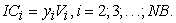(17)

At the beginning, the same voltage is assumed for all buses, load currents and capacitive currents are calculated for all the equations (16) and (17). The active and reactive power loss are also calculated from the equations (18) and (19):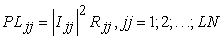(18)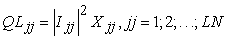(19)

jj: number of branches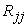: branch resistant of jj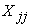: branch reactance of jj.

3.1. Backward-forward Sweep Load Flow Algorithm

1. The voltage of all buses is selected as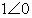pu.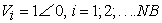(20)

2. The active and reactive power in each load buses are calculated based on the load model of the equations (1) and (2).

3. The load current in the bus i is calculated according to the corresponding load model from equation (16).

4. Backward sweep: Moving from the end of the buses to the base bus, the flow of the branches is calculated from the following equation.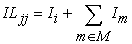(21)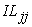: Branch current of jj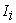: Current injected by load connected to bus i

M: Branches sets connected to jj in bus i

m: The branch number connected to the branch jj at bus i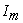: Branch current (flow) of m.

Since the active and reactive power in the load model is voltage dependent and each new voltage is calculated, the load current and load power in each iteration must be calculated.

5. forward sweep: After obtaining the current of branches from the backward sweep and moving from the base bus to the end of the bus, the voltages of buses are obtained from the following equation.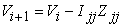(22)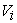: The primary bus voltage of branch jj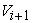: The End bus voltage of branch jj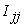: Branch current of branch jj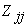: Impedance of the branch jj.

6- This process continues as long as the maximum difference of bus voltages (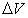) in two successive repetitions is exceeded of the given accuracy (ε). Flowchart of the load flow algorithm is shown in Figure 2.

• Figure 2. Load flow flowchart of backward-forward sweep

### 4. The Effect of Load Modeling on Load Flow Results in Distribution Networks-Part 1: Losses

4.1. Load Flow in 10-bus Network

The first network is a distribution network of 10 buses 23 kV, whose data was taken from 18. α, β and γ are the load contribution percentage of constant impedance, constant current and constant power and the ZIP equation is considered as equations (6) and (7). The 10-bus single-line diagram is given in Figure 3.

• Figure 3. 10-bus single-line diagram

4.1.1. Power loss with Constant Power Load Model in 10-bus Network

For the 10-bus network with the given data, the load is considered as constant power, and the results of the active power loss generated in the lines are shown in Figure 4. The total power loss in active power is 783.4347 kW. The maximum active power loss occurs in branch 5 and is equal to 190.1983 kW.

4.1.2. Power Loss with Constant Current Load Model in 10-bus Network

Here, for 10-bus network, the load model is considered as a constant current. the results of the active power loss generated in the lines are shown in Figure 5. The total active power loss is 610.66 kW. The maximum active power loss occurs in the branch 5 and is equal to 147.2024 kw.

4.1.3. Power Loss with Constant Impedance Load Model in 10-bus Network

Like the constant power and current load model, the constant impedance load model is considered for the 10-bus network, load flow is done and the results of the active power loss generated in the lines are shown in Figure 6. The total active power loss is 503.05 kW. The maximum active power loss occurs in branch 3 with a value of 124.8866 kW.

• Figure 4. Active power loss on 10-bus network lines with constant power load model
• Figure 5. Active power loss on 10-bus network lines with constant current load model
• Figure 6. Active power loss in 10-bus network lines with constant impedance load model

The total active and reactive power loss generated in 10-bus network lines for constant power, constant current and constant impedance load models are given in Table 4.

4.1.4. Power Loss with the ZIP Load Model in the 10-bus Network

As mentioned in section 2, the ZIP model is one of the most commonly used polynomial models widely used in distribution network studies. Here, for several types of ZIP models with different percentages, load flow is performed, and the results of active and reactive power loss are shown in Table 5. The ZIP model used in the load flow is the model mentioned in equations (6) and (7).

For the ZIP model, which is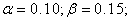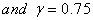, the total power loss is equal to 717.1355 kW and the total reactive power loss is equal to 960.1059 kVar, and for the ZIP model, which is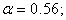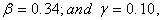the total active power loss is 556.6909 kW and the total reactive power loss is 771.8249 kVar. Based on Table 5, the higher the percentage of constant impedance load, the lower the power loss.

4.2. 30 bus distribution network

The 10-bus distribution network is a network with a main line and no lateral branch. Therefore, in order to ensure the effect of load modeling on the load flow results and the realization of the discussion, the load flow is also performed on a 30-bus network. single-line diagram of 30-bus distribution network whose data is taken from 14 has plotted in Figure 7. For this network, like the 10-bus network, for constant power, constant current, constant impedance and ZIP load models, the load flow is performed and the results of power loss and voltage profiles are achieved.

• Figure 7. 30-bus single-line diagram

4.2.1. Active Power Loss with Constant Power Load Model in 30-bus Network

For the 30-bus network with the given data, load flow is performed and active power losses are shown in Figure 8. The total active loss is 874.3309 kW.

4.2.2. Power loss with Constant Current Load Model in 30-bus Network

The active power loss of the 30-bus network lines for the constant current model are shown in Figure 9. The total active loss is 723.9910 kW.

4.2.3. Power Loss with Constant Impedance Load Model in 30-bus Network

Considering the constant impedance load model, active power loss is shown in Figure 10. The total active power loss is 618.6203 kW.

Table 6 shows total active power loss and total reactive power consumption in constant power, constant current and constant impedance load models in a 30-bus network. It is clear from the results that the constant impedance model has lower power loss.

• Figure 8. Active power loss on 30-bus network lines with constant power load model
• Figure 9. Active power loss on Power loss with constant load impedance model in 30-bus network
• Figure 10. Effective power loss in 30-bus network lines with constant impedance load model

4.2.4. Power Loss with the ZIP Load Model in the 30-Bus Network

In the 30-bus network for the ZIP-load model with the values of α, β and γ, load flow is also performed and the results of active and reactive power loss for each model are shown in Table 7. For the ZIP model, which is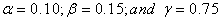, the total active power loss is 817.7726 kW, and the total reactive power loss is equal to 239.6968 kVar and for the ZIP model, which is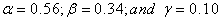, the total active power loss is 673.3070 kW and the total reactive power loss is 198.0415 kVar.

### 5. The Effect of Load Modeling on Load Flow Results in Distribution Networks- Part 2: Voltage Profile

5.1. Voltage Profile in the 10-bus Distribution Network
5.1.1. Voltage Profile of the Constant Power Load Model in the 10-bus Network

Load flow for the 10-bus network with a constant power load model is performed and the voltage profile in the network buses in Figure 11 is shown. The lowest voltage is for bus 10, which is 0.8375.

5.1.2. Voltage Profile of the constant Current Load Model in the 10-bus Network

In the 10-bus network, the results of the network bus voltage for the constant current load model are shown in Figure 12. The minimum network voltage in the constant current load model is equal to 0.8581 in bus 10.

5.1.3. Voltage Profile of the Constant Impedance Load Model in the 10-bus Network

In the 10-bus network, the results of the network bus voltages for the constant impedance load model are shown in Figure 13. The minimum network voltage in the constant impedance load model is 0.8722 in the bus 10.

The minimum voltage for constant power, constant current and constant impedance models in the 10-buss network is shown in Table 8.

• Figure 11. Bus voltage at 10-bus network with constant power load model
• Figure 12. Bus voltage at 10-bus network with constant current load model
• Figure 13. Bus voltage at 10-bus network with constant impedance load model

For all three models, the minimum voltage has occurred in bus 10.

5.1.4. The Voltage Profile of the ZIP Load Model in the 10-bus Network

The results for the Minimum voltage in each ZIP model are shown in Table 9. For the ZIP model, which has a value ofand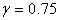the minimum bus voltage is equal to 0.8432. For a ZIP model with values of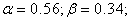and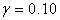the minimum voltage is 0.8591. As it is shown when the constant power load model factor (i.e. γ) approaches to 1, the voltage level is lower, and vice versa, whatever the constant current load model factor (i.e. β) and the constant impedance load model factor (i.e. α) are closer to one, the voltage level will be higher.

5.2. The Voltage Profile in the 30-bus Network
5.2.1. Voltage Profile of the Constant Power Load Model in the 30-bus Network

For the 30-bus network with the given data, the load flow is performed and the voltages of the buses for the constant power load model are shown in Figure 14. The lowest network voltage in the constant power load model is 0.8831 per unit in the bus 27.

5.2.2. Voltage Profile of the Constant Current Load Model in the 30-bus Network

Figure 15 shows the voltage profile in a 30-bus distribution network with constant current load models after performing load flow, with a minimum network voltage of 0.8943 in bus 27.

5.2.3. The Voltage Profile of the Constant Impedance Load Model in the 30-bus Network

The 30-bus network voltage profile in the presence of constant impedance load model is shown in Figure 16. The minimum network voltage is 0.9028 in bus 27.

• Figure 14. Bus voltage at 30-bus network with constant power load model
• Figure 15. Bus voltage at 30-bus network with constant current load model
• Figure 16. Bus voltage at 30-bus network with constant impedance load model

The minimum voltage for constant power, constant current and constant impedance models in the 30-bus network is shown in Table 10. For all three models, the minimum voltage load has occurred in bus 27.

5.2.4. The Voltage Profile of the ZIP Load Models in the 30-bus Network

The load flow of the 30-bus network is done in the presence of the ZIP load models, where the values of α, β and γ for the models are shown in Table 11. The results for the lowest voltage in each model are shown in Table 11. For the ZIP model, which has a value of, the minimum bus voltage is equal to 0.8872. For a ZIP model with values of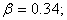and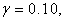the minimum voltage is 0.8984. As you can see, the constant power load model factor (i.e. γ) is close to one, the voltage level is lower and, vice versa, the constant current load model factor (i.e. β) and the constant impedance load model factor (α) is closer to one, the voltage level will be higher.

### 6. Conclusion

The design and development of distribution networks requires a comprehensive study. In this process, the simulation of the network and the modeling of its constituent elements is a prerequisite. One of the important elements in distribution networks is network loads that should be modeled correctly and fully. Currently, in most studies of distribution networks, such as the optimal placement of capacitor banks and their size, the optimal network reconfiguration, the optimal location of voltage regulators, the optimal location of distributed generation sources, etc., the constant power model for load modeling is used. While the grid is composed of different types of load, the lack of accurate modeling can lead to wrong and non-optimal results. Because load flow is the basis of any of the above studies the effect of load modeling on the results of load flow can reflect the impact of load modeling on other distribution network studies. In this paper, describing the load flow method of backward-forward sweep and load models, the effect of load modeling on the results of load flow was investigated. For two 10-bus and 30-bus distribution networks, a backward-forward sweep load flow method was perfomed and tested for constant power, constant current, constant impedance, and ZIP load models. Paying attention to the diagrams and comparing the results of the load flow, shows that exact load models should be used in distribution network studies to obtain precise and accurate solutions. Using a constant power load model, which is common to the distribution network studies, cannot result in precise and optimal solutions.

### References

  W.D. Stevenson., Elements of Power System Analysis, McGraw-Hill, 1982. In article View Article  W.G. Tinney, C.E. Hart., Power Flow Solutions by Newton’s Method, IEEE Transaction on Power Apparatus Systems, Vol. 86, pp. 1449-1457, 1967. In article View Article  B. Stott, O. Alsac., Fast Decoupled Load Flow, IEEE Trans. Power Apparatus Systems, Vol. 93, No. 3, pp. 859-869, 1974. In article View Article  S. Iwamoto, Y. Tamura., A Load Flow Calculation Method for Ill-Conditioned Power Systems, IEEE transaction on power apparatus, Vol. 100, No. 4, pp. 1736-1743, 1981. In article View Article  D. Rajicic, Y. Tamura., A Modification to Fast Decoupled Power Flow for Networks with High R/X Ratios, IEEE Transaction on Power System, Vol. 3, No. 2, pp. 743-746, 1988. In article View Article  G.W. Stagg, A.H. El-Abiad., Computer Methods in Power System Analysis, McGraw-Hill, 1968. In article  W. H. Kersting., A Method to Teach the Design and Operation of a Distribution System, IEEE Transactions on Power Apparatus and Systems, Vol.103, No.7, pp. 1945-1952, 1999. In article View Article  R. A. Stevens, D. T. Rizy, S. L. Purucker., Performance of Conventional Power Flow Routines for Real Time Distribution Automation Application, Proceedings of 18th southeastern symposium on system theory, pp. 196-200, April 1986. In article View Article  S. Ghosh, D. Das., Method for Load-Flow Solution of Redial Distribution Networks, IEEE Proc-Gener. Transm. Distrib. Vol.146, No. 6, pp.641-648, November 1999. In article View Article  D. Shirmohammadi, H.W. Hong, A. Semlyen, G.X. Luo., A Compensation-Based Power-Flow Method for Weakly Meshed Distribution and Transmission Networks, IEEE Transaction on Power Systems, Vol. 3, No. 2, pp. 753-762, 1988. In article View Article  J. H. Teng., A Network-Topology-Based Three-Phase Load Flow for Distribution Systems, Proceeding National Science Council, Republic of China, Vol. 24, No. 4, pp. 259-264, 2000. In article View Article  J. H. Teng., A Direct Approach for Distribution System Load Flow Solutions, IEEE Transaction on Power Delivery, Vol. 18, No. 3, pp. 882-887, 2003. In article View Article  S. Mok, S. Elangovan, C. Longjian, M. Salama., A New Approach for Power-Flow Analysis of Balanced Radial Distribution Systems, Electric Machines Power System, Vol. 28, pp. 325-340, 2000. In article View Article  U. Eminoglu, M. H. Hocaoglu., A New Power Flow Method for Radial Distribution Systems Including Voltage Dependent Load Models, Electrical Power System Research, Vol.76, pp. 106-114, 2005. In article View Article  Jia Hou, Zhao Xu, Zhao Yang Dong, load Modeling Practice in a Smart Grid Environment, IEEE Conference, 2011. In article View Article  K. Qian, Ch. Zhou,M. Allan,Y. Yuan., Effect of Load Models on Assessment of Energy Losses in Distributed Generation Planning, Electrical Power Energy Systems, Vol. 33,pp. 1243-1250, 2011. In article View Article  P. kundur., Power System Stability and Control, second ed., Wiley, New York, pp. 1-1166, 1994. In article View Article  Mohamed M. Hamada, Mohamed A. A. Wahab, Abou-Hashema M.El-Sayed, Husms. Ramadan., A Proposed Strategy for Capacitor Allocation in Radial Distribution Feeders, IEEE Proc. Int. Conf. pp146-151, 2008. In article PubMed

Published with license by Science and Education Publishing, Copyright © 2018 Nour ali Rostami and Mahmoud Oukati SadeghThis work is licensed under a Creative Commons Attribution 4.0 International License. To view a copy of this license, visit http://creativecommons.org/licenses/by/4.0/